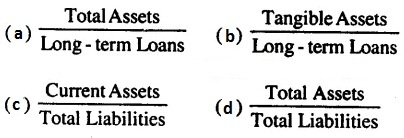# Class 12 Accountancy MCQ Questions of Accounting Ratios with Answers

274 views
in Accounts
closed

Class 12 Accountancy MCQ Questions of Accounting Ratios with Answers?

+1 vote
by (12.3k points)
edited by

Class 12 Accountancy MCQ Questions of Accounting Ratios with Answers are available here. MCQ Questions for Class 12 Accountancy with Answers were Prepared Based on Latest Exam Pattern and schedule. Our specialists have planned MCQ Questions for Class 12 Accountancy with Answers for all sections in your NCERT Class 12 Accountancy book.

Improve your preparation with MCQ on Accounting Ratios Class 12 Objective Questions. You can likewise confirm your answers from our gave Accounting Ratios Class 12 MCQ Questions with Answers. Realize your preparation level on MCQ Questions for Class 12 Accountancy with Answers. Solving the Multiple Choice Questions for Class 12 Accountancy is the simplest method to comprehend the ideas. Practice Accountancy MCQ Questions with Answers without carelessness to score great marks in the exam.

1. Two basic measures of liquidity are :

(A) Inventory turnover and Current ratio
(B) Current ratio and Quick ratio
(C) Gross Profit ratio and Operating ratio
(D) Current ratio and Average Collection period

2. Current Ratio is :

(A) Solvency Ratio
(B) Liquidity Ratio
(C) Activity Ratio
(D) Profitability Ratio

3. Current Ratio is :

(A) Liquid Assets/Current Assets
(B) Fixed Assets/Current Assets
(C) Current Assets/Current Liabilities
(D) Liquid Assets/Current Liabilities

4. Liquid Assets do not include :

(A) Bills Receivable
(B) Debtors
(C) Inventory
(D) Bank Balance

5. Ideal Current Ratio is :

(A) 1 : 1
(B) 1 : 2
(C) 1 : 3
(D) 2 : 1

6. Working Capital is the :

(A) Cash and Bank Balance
(B) Capital borrowed from the Banks
(C) Difference between Current Assets and Current Liabilities
(D) Difference between Current Assets and Fixed Assets

7. The formula for ascertaining Total Assets to Debt Ratio is8. Proprietory Ratio indicates the relationship between proprietor’s funds and….

(a) Reserve
(b) Share Capital
(c) Total Assets
(d) Debentures

9. Proprietory ratio is calculated by the following formula:10. Which one of the following ratios is most important in determining the long-term solvency of a company ?

(a) Profitability Ratio
(b) Debt-Equity Ratio
(c) Stock Turnover Ratio
(d) Current Ratio

11. Total Assets ₹ 8,10,000 Total Liabilities ₹ 2,60,000 Current Liabilities ₹ 40,000 Debt-equity ratio is:

(a) 0.05 : 1
(b) 0.4 : 1
(c) 2.5 : 1
(d) 4 : 1

12. Equity share capital ₹ 15,00,000 Reserve and Surplus ₹ 7,50,000 Total Assets ₹ 45,00,000 Properletory Ratio ?

(a) 50%
(b) 33.3%
(c) 200%
(d) 60%

13. Total Assets ₹ 7,70,000 Total Liabilities ₹ 2,60,000 Current Liabilities ₹ 40,000 Total Assets to Debt Ratio is:

(a) 3.5 : 1
(b) 2.56 : 1
(c) 2.8 : 1
(d) 3 : 1

14. Profitability Ratios are generally expressed in :

(a) Simple Ratio
(b) Percentage
(c) Times
(d) None of these

15. Proprietary or equity ratio is equal to

a) Shareholders funds/ total assets
b) Shareholders funds+ total assets
c) Shareholders funds- total assets
d) None of the options

16. Long term solvency is indicated by :

a) Current Ratio
b) Quick Ratio
c) Net Profit Ratio
d) Debt/Equity Ratio

17. Two basic measures of liquidity are:

a. Inventory turnover and Current ratio
b. Current ratio and Quick ratio
c. Gross Profit ratio and Operating ratio
d. Current ratio and average Collection period

18. Accounting ratios are an important tool of

a) Financial statement analysis

b) Trial Balance

c) Financial statement analysis and Trial Balance

d) None of the options

19. The solvency position of any firm is determined and measured with the help of

a) None of the options
b) Activity Ratios
c) Profitability Ratios
d) Solvency ratios

20. Management are interested in

a) Activity Ratios and Profitability Ratios
b) Activity Ratios
c) Profitability Ratios
d) None of the options

21. The ________ ratios are primarily measures of return :

(a) liquidity
(b) activity
(c) debt
(d) profitability

22. The excess of Current ratio is also treated as a sign of managerial

a) Inefficiency and Efficiency
b) Efficiency
c) Inefficiency
d) None of the options

23. Liquid Assets do not include:

a. Bills Receivable
b. Debtors
c. Inventory
d. Bank Balance

24. When the concept of ratio is defined in respected to the items shown in the financial statements, it is termed as

a) Accounting ratio

b) Financial ratio

c) Costing ratio

d) None of the options

25. Which ratio is considered as safe margin of solvency?

a) Current ratio

b) Liquid ratio

c) Current ratio

d) None of the options

+1 vote
by (12.3k points)

1. Answer (B) Current ratio and Quick ratio

2. Answer (B) Liquidity Ratio

3. Answer (C) Current Assets/Current Liabilities

4. Answer (C) Inventory

5. Answer (D) 2 : 1

6. Answer (C) Difference between Current Assets and Current Liabilities8. Answer (c) Total Assets10. Answer (b) Debt-Equity Ratio

11. Answer (c) 2.5 : 1

12. Answer (a) 50%

13. Answer (a) 3.5 : 1

14. Answer (b) Percentage

15. Answer (c) Shareholders funds- total assets

16. Answer (d) Debt/Equity Ratio

17. Answer (b) Current ratio and Quick ratio

18. Answer (c) Financial statement analysis and Trial Balance

19. Answer (d) Solvency ratios

20. Answer (a) Activity Ratios and Profitability Ratios

21. Answer (d) profitability

22. Answer (c) Inefficiency

23. Answer (c) Inventory

24. Answer (a) Accounting ratio

25. Answer (a) Current ratio